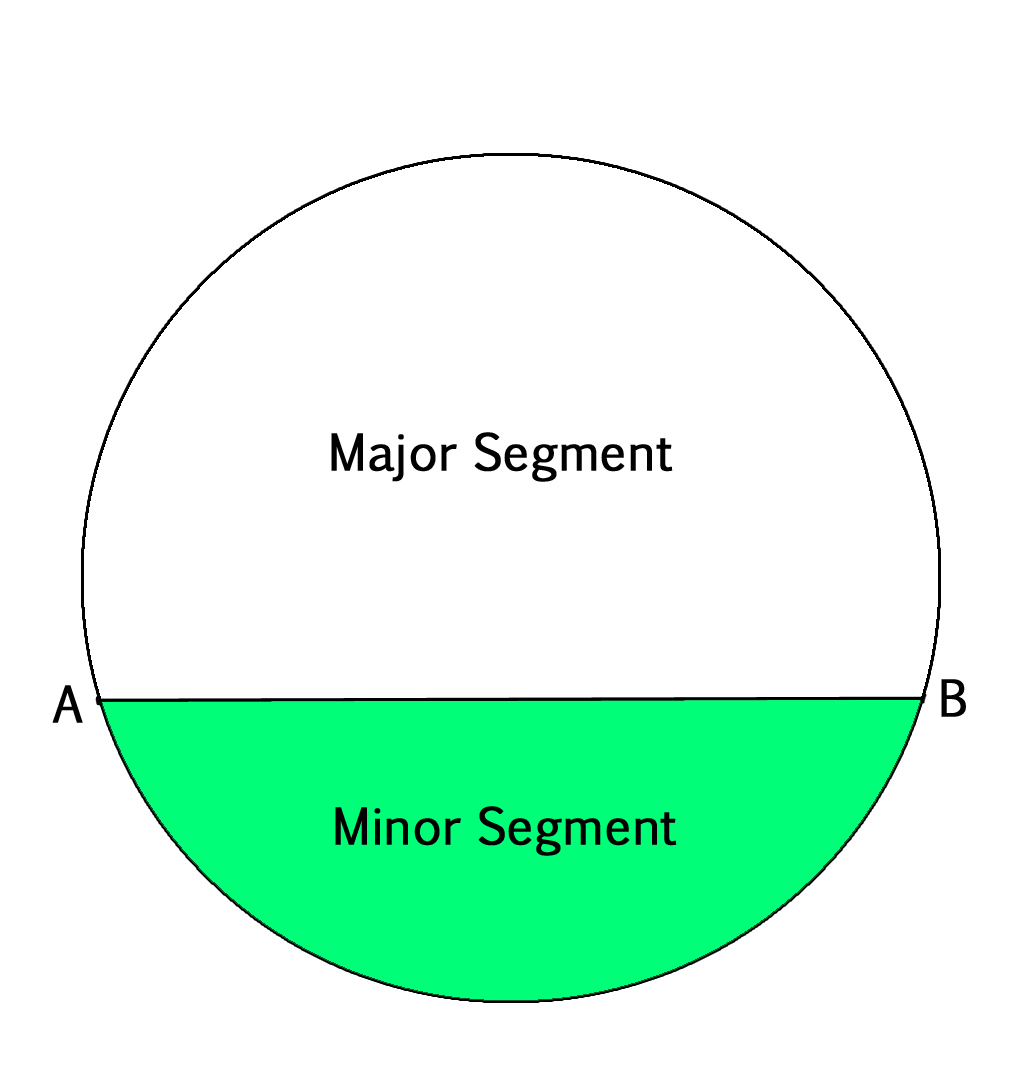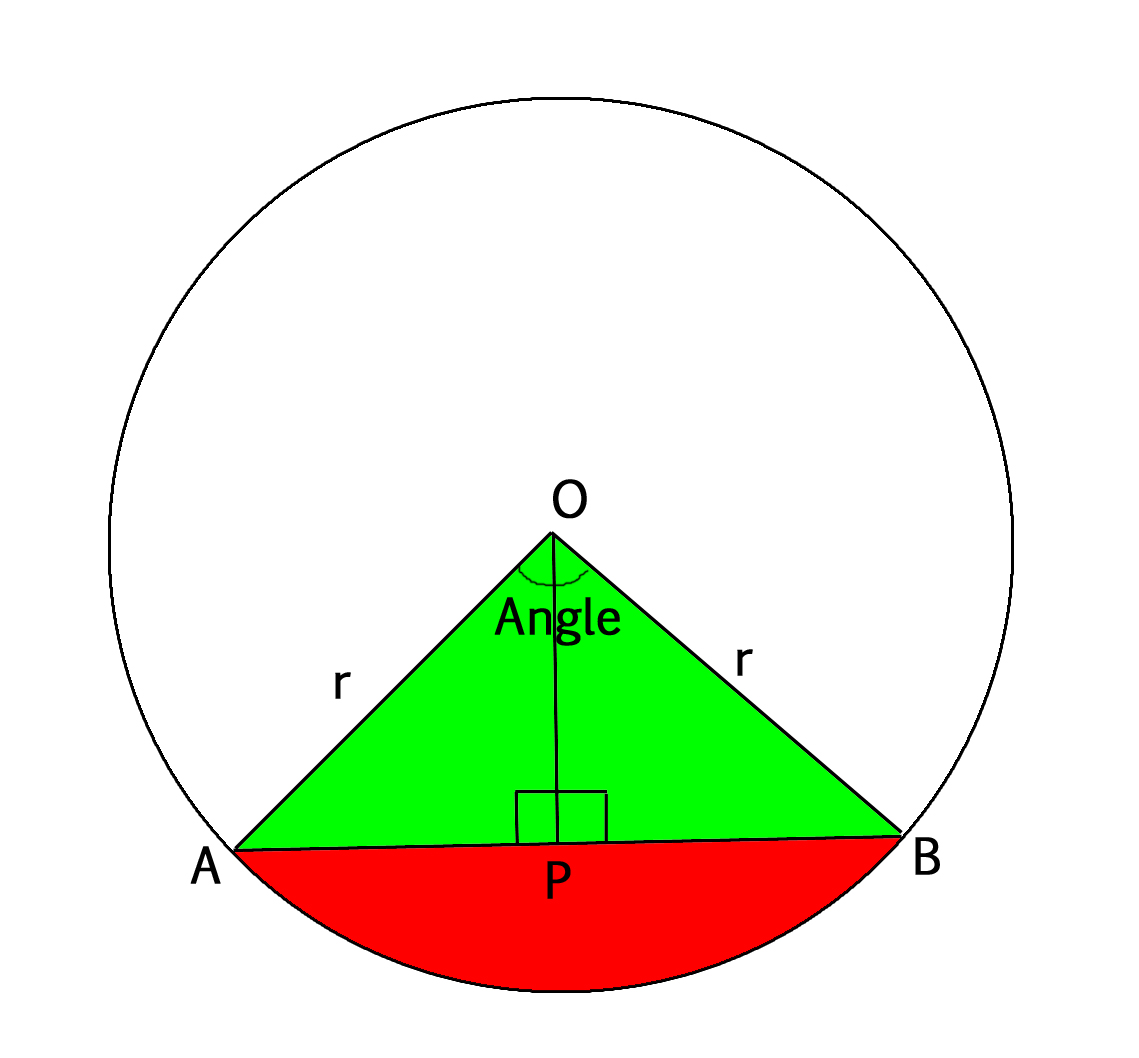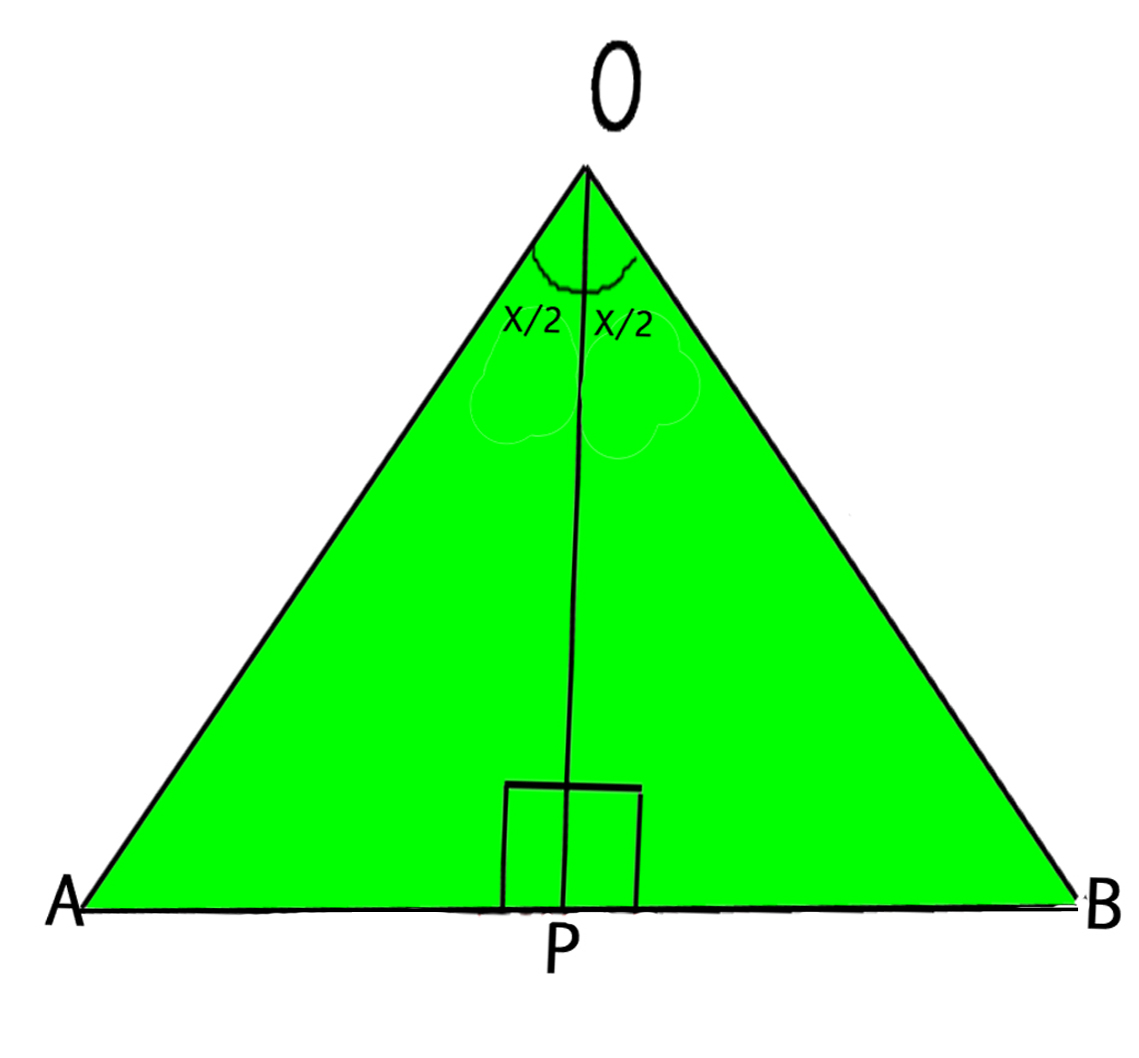# Program to find area of a Circular Segment

In a circle, if a chord is drawn then that chord divides the whole circle into two parts. These two parts of the circle are called segments of the circle. The smaller area is known as the Minor segment and the larger area is called as the Major segment.

In the figure below, the chord AB divides the circle into minor and major segments.We are given radius of circle and angle that forms minor segment. We need to find areas of two segments.

Examples :

```Input :
angle = 120.0
Output :
Area of minor segment 270.855
Area of major segment 1114.59

Input :
angle = 90.0
Output :
Area of minor segment 28.5397
Area of major segment 285.619
```

## Recommended: Please try your approach on {IDE} first, before moving on to the solution.

#### Area of the segment :

For that, we join the end points of the chord with the center of the circle resulting in a sector which subtends some ‘angle’ at the center. And a perpendicular is drawn from the center of the circle on the chord AB. By congruence of triangles, we obtain that the ∠ AOP = ∠ BOP = 1/2(angle).Formula for Area of Segment :

```Area of Segment = Area of sector - Area of Triangle OAB
= pi * r2 * (angle/360) -
Area of Triangle OAB```

For detailed information about formula of Area of Sector, refer https://www.geeksforgeeks.org/area-of-a-sector/.```In the figure above, assume angle made by sector = X,
so ∠ AOP = ∠ BOP = X/2

Area of Triangle AOB = 1/2 * base * height
= 1/2 * AB * OP

Now in Triangle AOP, By trigonometry
Cos(X/2) = OP/AO  i.e. OP = AO * Cos(X/2)
OP = r * Cos(X/2)
Sin(X/2) = AP/AO  i.e. AP = AO * Sin(X/2)
AP = r * Sin(X/2)

So,
Base = AB = AP + PB
= 2 * AP
= 2 * r * Sin(X/2)

Height = OP = r * Cos(X/2)

Area of triangle = 1/2 * (2 * r * Sin(X/2)) * (r * Cos(X/2))
= 1/2 * r2 * Sin(X)
[Using identity 2 * Sin(A) * Cos(A)]
= Sin(2 * A))

Hence Area of Segment =  pi * r2 * (angle/360) - 1/2 * r2 * Sin(angle)
```

## C++

 `// C++ Program to  ` `// find area of  ` `// segment of a ` `// circle ` `#include ` `using` `namespace` `std; ` ` `  `float` `pi = 3.14159; ` ` `  `// Function to find ` `// area of segment ` `float` `area_of_segment(``float` `radius, ` `                      ``float` `angle) ` `{ ` `    ``// Calculating area of sector ` `    ``float` `area_of_sector = pi * ` `                           ``(radius * radius)  ` `                           ``*(angle / 360); ` ` `  `    ``// Calculating area of triangle ` `    ``float` `area_of_triangle = (``float``)1 / 2 * ` `                             ``(radius * radius) * ` `                             ``sin``((angle * pi) / 180); ` ` `  `    ``return` `area_of_sector - area_of_triangle; ` `} ` ` `  `// Driver Code ` `int` `main() ` `{ ` `    ``float` `radius = 10.0, angle = 90.0; ` `    ``cout << ``"Area of minor segment = "` `        ``<< area_of_segment(radius, angle) << endl; ` ` `  `    ``cout << ``"Area of major segment = "` `        ``<< area_of_segment(radius, (360 - angle)); ` `} `

## Java

 `// Java Program to find area of ` `// segment of a circle ` `class` `GFG { ` `static` `float` `pi = ``3``.14159f; ` ` `  `static` `float` `area_of_segment(``float` `radius, ` `                              ``float` `angle) ` `{ ` `    ``// Calculating area of sector ` `    ``float` `area_of_sector = pi *  ` `   ``(radius * radius) * (angle / ``360``); ` ` `  `    ``// Calculating area of triangle ` `    ``float` `area_of_triangle = ` `        ``(``float``)``1` `/ ``2` `* (radius * radius) * ` `        ``(``float``)Math.sin((angle * pi) / ``180``); ` ` `  `    ``return` `area_of_sector - area_of_triangle; ` `} ` ` `  `// Driver Function ` `public` `static` `void` `main(String[] args)  ` `{ ` `    ``float` `radius = ``10``.0f, angle = ``90``.0f; ` `    ``System.out.println(``"Area of minor segment = "` `+ ` `                    ``area_of_segment(radius, angle)); ` ` `  `    ``System.out.println(``"Area of major segment = "` `+ ` `                    ``area_of_segment(radius, (``360` `- angle))); ` `} ` `} ` ` `  `// This code is contributed by Anant Agarwal. `

## Python

 `# Python3 Program  ` `# to find area of ` `# segment of a ` `# circle ` `import` `math ` ` `  `pi ``=` `3.14159` ` `  `# Function to find ` `# area of segment ` `def` `area_of_segment(radius, angle): ` `    ``# Calculating area of sector ` `    ``area_of_sector ``=` `pi ``*`  `                     ``(radius ``*` `radius) ` `                     ``*` `(angle ``/` `360``) ` ` `  `    ``# Calculating area of triangle ` `    ``area_of_triangle ``=` `1` `/` `2` `*`  `                       ``(radius ``*` `radius) ``*` `                       ``math.sin((angle ``*` `pi) ``/` `180``) ` ` `  `    ``return` `area_of_sector ``-` `area_of_triangle; ` ` `  ` `  `# Driver Code ` `radius ``=` `10.0` `angle ``=` `90.0` `print``(``"Area of minor segment ="``, ` `       ``area_of_segment(radius, angle)) ` `print``(``"Area of major segment ="``, ` `      ``area_of_segment(radius, (``360` `-` `angle))) ` `       `  `      `  `# This code is contributed by Smitha Dinesh Semwal `

## C#

 `// C# Program to find area  ` `// of segment of a circle ` `using` `System; ` ` `  `class` `GFG { ` `      ``static` `float` `pi = 3.14159f; ` ` `  `      ``static` `float` `area_of_segment(``float` `radius, ` `                                   ``float` `angle) ` `{ ` `    ``// Calculating area of sector ` `    ``float` `area_of_sector = pi * (radius * radius)  ` `                             ``* (angle / 360); ` ` `  `    ``// Calculating area of triangle ` `    ``float` `area_of_triangle =(``float``)1 / 2 * (radius * radius)  ` `                            ``*(``float``)Math.Sin((angle * pi) / 180); ` ` `  `    ``return` `area_of_sector - area_of_triangle; ` `} ` ` `  `    ``// Driver Function ` `    ``public` `static` `void` `Main()  ` `{ ` `    ``float` `radius = 10.0f, angle = 90.0f; ` `    ``Console.WriteLine(``"Area of minor segment = "` `+ ` `                        ``area_of_segment(radius, angle)); ` ` `  `    ``Console.WriteLine(``"Area of major segment = "` `+ ` `                       ``area_of_segment(radius, (360 - angle))); ` `} ` `} ` ` `  `// This code is contributed by vt_m. `

## PHP

 ` `

Output :

```Area of minor segment = 28.5397
Area of major segment = 285.619
```

Attention reader! Don’t stop learning now. Get hold of all the important DSA concepts with the DSA Self Paced Course at a student-friendly price and become industry ready.

My Personal Notes arrow_drop_upCheck out this Author's contributed articles.

If you like GeeksforGeeks and would like to contribute, you can also write an article using contribute.geeksforgeeks.org or mail your article to contribute@geeksforgeeks.org. See your article appearing on the GeeksforGeeks main page and help other Geeks.

Please Improve this article if you find anything incorrect by clicking on the "Improve Article" button below.

Improved By : vt_m